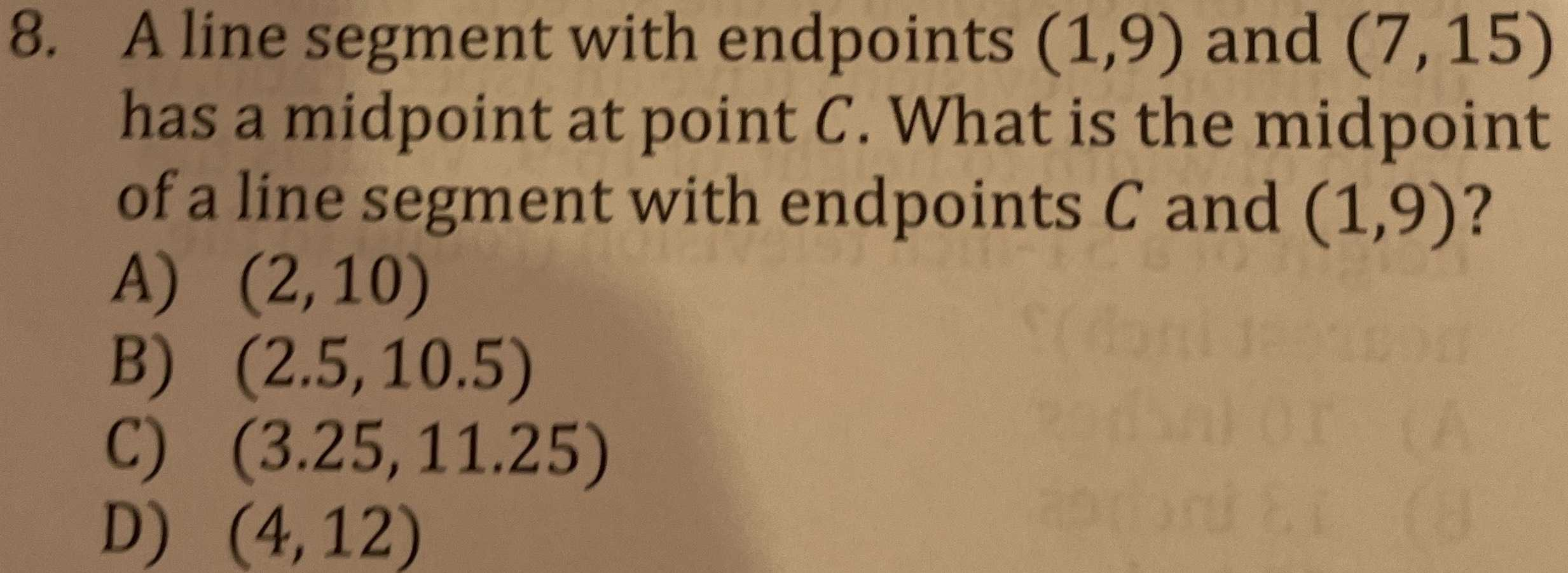### ¿Todavía tienes preguntas de matemáticas?

Pregunte a nuestros tutores expertos
Algebra
Pregunta8. A line segment with endpoints $$( 1,9 )$$ and $$( 7,15 )$$ has a midpoint at point $$C$$ . What is the midpoint of a line segment with endpoints $$C$$ and $$( 1,9 )$$ ?

A) $$( 2,10 )$$

B) $$( 2.5,10.5 )$$

C) $$( 3.25,11.25 )$$

D) $$( 4,12 )$$